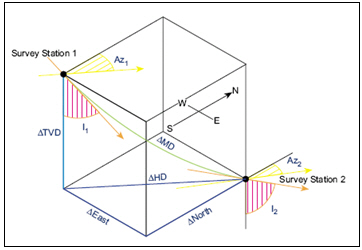## Dogleg Severity Calculation Based on Tangential Method

From the previous post, I posted about how to calculate dogleg severity based on Radius of Curvature Method. What’s more, there is another way to calculate dogleg severity based on the concept of Tangential Method.

The following formula provides dogleg severity in degrees/100 ft and is based on the Tangential Method:

Dogleg severity (DLS) = 100 ÷ {MD x [(sin I1 x sin I2) x (sin Az1 x sin Az2 + cos Az1 x cos Az2) + (cos I1 x cos I2)]}

where

DLS = dogleg severity in degrees/l00 ft

MD = measured depth between survey points, ft

I1 = inclination (angle) at upper survey in degrees

I2 = inclination (angle) at lower in degrees

Az1= Azimuth direction at upper survey

Az2 = Azimuth direction at lower survey

Calculation example for dogleg severity based on Tangential Method

Survey 1

Depth = 7500 ft

Inclination = 45 degree (I1)

Azimuth = 130 degree (Az1)

Survey 2

Depth = 7595 ft

Inclination = 52 degree (I2)

Azimuth = 139 degree (Az2)

Dogleg severity (DLS) = 100 ÷ {95 x [(sin 45 x sin 52) x (sin 130 x sin 139 + cos 130 x cos 139) + (cos 45 x cos 52)]}

Dogleg severity (DLS) = 1.07 degree/100 ft

Please find the Excel sheet for calculating dogleg severity with the concept of Tangential Method

## Dogleg Severity Calculation based on Radius of Curvature Method

Dogleg severity (DLS) is a normalized estimation, normally described in degrees per 100 feet or degree per 30 meters, of the overall well bore curvature between two consecutive directional surveys. Regarding a planned well path, dogleg severity may be synonymous about build and/or turn. The following formula provides dogleg severity in degrees/100 ft  based on the Radius of Curvature Method.Dogleg severity (DLS) = {cos-1 [(cos I1 x cos I2) + (sin I1 x sin I2) x cos (Az2 – Az1)]} x (100 ÷ MD)

Where;

DLS = dogleg severity in degrees/l00 ft

MD = Measured Depth between survey points in ft

I1 = Inclination (angle) at upper survey in degrees

I2 = Inclination (angle) at lower in degrees

Az1= Azimuth direction at upper survey

Az2 = Azimuth direction at lower survey

Example for dogleg severity based on Radius of Curvature Method

Survey 1

Depth = 7500 ft

Inclination = 45 degree (I1)

Azimuth = 130degree (Az1)

Survey 2

Depth = 7595 ft

Inclination = 52 degree (I2)

Azimuth = 139 degree (Az2)

Dogleg severity (DLS) = {cos-1 [(cos 45 x cos 52) + (sin 45 x sin 52) x cos (139 – 130)]} x (100 ÷ 95)

Dogleg severity (DLS) =  10.22 degree/100 ft

Please find the Excel sheet used to calculate dogleg severity based on Radius of Curvature Method.